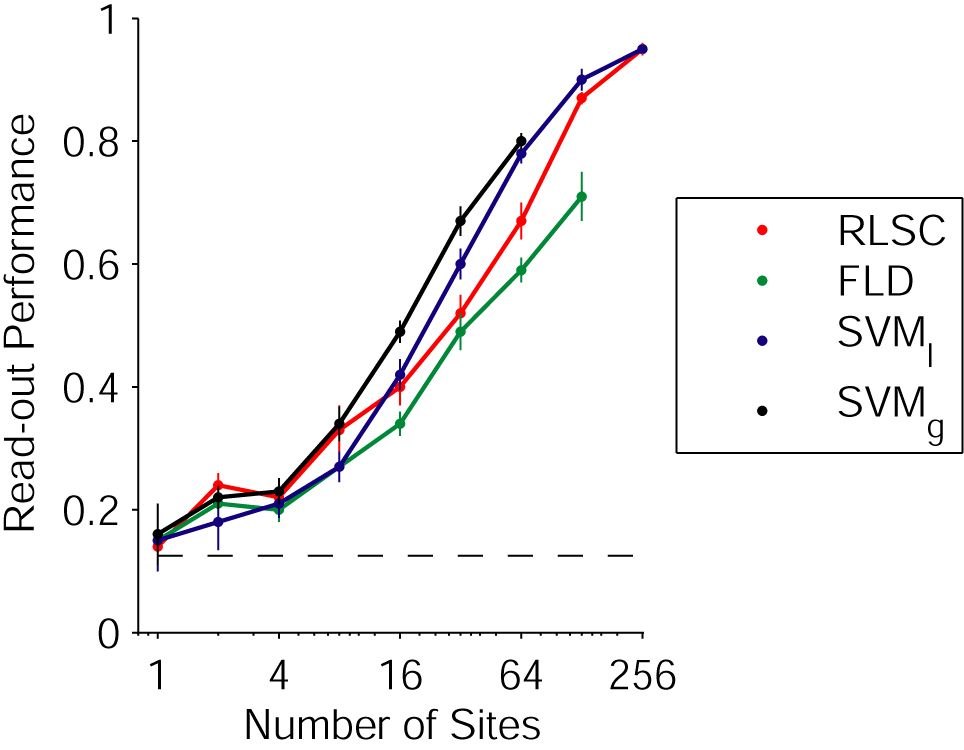Comparison of different statistical classifiers

Comparison of the classification performance (for the categorization task) results using different statistical classifiers: regularized least squares classifier (RLSC, red), Fisher linear discriminant (FLD, green), support vector machine with linear kernel (SVM_l, blue), support vector machine with gaussian kernel (SVM_g, black).Parameters

signal = MUA, bin size = 50 ms, time interval = [100;300) ms
RLSC, nu = 10
SVM_l, C=10
SVM_g, sigma=1

Top